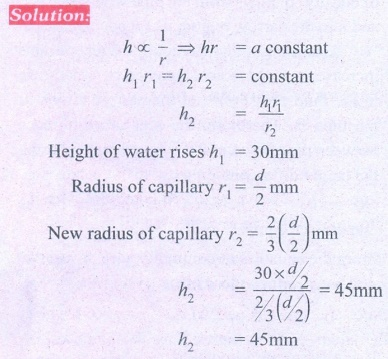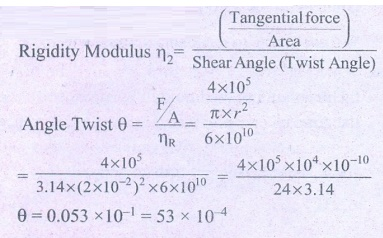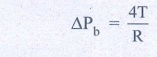Home | | Physics 11th std | Book Back Numerical Problems

# Book Back Numerical Problems

Physics : Properties of Matter : Book Back Exercise, Example Numerical Question with Answers, Solution : Book Back Numerical Problems

## Numerical Problems

### 1. A capillary of diameter dmm is dipped in water such that the water rises to a height of 30mm. If the radius of the capillary is made (2/3) of its previous value, then compute the height up to which water will rise in the new capillary?(Answer: 45 mm)2. A cylinder of length 1.5ŌĆåm and diameter 4 cm is fixed at one end. A tangential force of 4 ├Ś 105 N is applied at the other end. If the rigidity modulus of the cylinder is 6 ├Ś 1010 NŌĆåm-2 then, calculate the twist produced in the cylinder.Solution:Length of a cylinder = 1.5 mDiameter = 4 cm; Tangential force F - 4 ├Ś 105NRigidity modulus ╬Ę = 6 ├Ś 1010 Nm-2Twist produced ╬Ė = ?╬Ė = 0.053 ├Ś 10-1 = 53 ├Ś 10-4(Answer: 45.60)3. A spherical soap bubble A of radius 2 cm is formed inside another bubble B of radius 4 cm. Show that the radius of a single soap bubble which maintains the same pressure difference as inside the smaller and outside the larger soap bubble is lesser than radius of both soap bubbles A and B.SolutionExcess pressure create with S.T of spherical surface of the liquid = ╬öP = 2T/RT - surface tensionIn case of soap bubbles,The excess pressure of air inside them is double due to the presence of two interfaces are inside and one outside.Excess pressure of air inside the bigger bubbleExcess pressure of air inside the smaller bubble 4s 4TAir pressure difference between the smaller bubble and the atmosphere will be equal toll sum of excess pressure inside the bigger smaller bubbles.Pressure different ╬öP = ╬öPb + ╬öPs= T + 2T = 3TExcess pressure inside a single soap bubble = 4T/R = 4T/4 = TPressure difference of single soap bubble less than radius of both T < 3T4. A block of Ag of mass x kg hanging from a string is immersed in a liquid of relative density 0.72. If the relative density of Ag is 10 and tension in the string is 37.12 N then compute the mass of Ag block. SoltionRelative density of liquid Žüliquid = 0.72Relative density of Ag ŽüAg = 10Mass of the Ag block = ?Tension in the string T = 37.12 NApparent weight Wapp = pAg - pliquid= 10-0.72 = 9.28m = TAg / gm = 37.12 / 9.28 = 4Mass x = 4 kg(Answer: x = 4 kg)5. The reading of pressure meter attached with a closed pipe is 5 ├Ś 105 NŌĆåm-2. On opening the valve of the pipe, the reading of the pressure meter is 4.5 ├Ś 105 Nm-2. Calculate the speed of the water flowing in the pipe.Solution(Answer: 10 ms-1)

Tags : Properties of Matter | Physics , 11th Physics : UNIT 7 : Properties of Matter
Study Material, Lecturing Notes, Assignment, Reference, Wiki description explanation, brief detail
11th Physics : UNIT 7 : Properties of Matter : Book Back Numerical Problems | Properties of Matter | Physics